# Diagonal + Pythagorean theorem - math problems

#### Number of problems found: 203

• Area of a rectangleCalculate the area of a rectangle with a diagonal of u = 12.5cm and a width of b = 3.5cm. Use the Pythagorean theorem.
• Cube diagonalDetermine the length of the cube diagonal with edge 37 mm.
• Body diagonalCalculate the length of the body diagonal of the 6cm cube.
• Diagonal of squareCalculate the side of a square when its diagonal is 10 cm.
• Body diagonalFind the cube surface if its body diagonal has a size of 6 cm.
• Square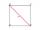Calculate the side of a square with a diagonal measurement 10 cm.
• Body diagonalCalculate the cube volume, whose body diagonal size is 75 dm. Draw a picture and highlight the body diagonal.
• Find diagonal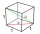Find the length of the diagonal of a cuboid with length=20m width=25m height=150m
• Body diagonalCalculate the volume and surface of the cube if the body diagonal measures 10 dm.
• Diagonal to areaCalculate the area of a rectangle in which the length of the diagonal is 10 cm.
• Square2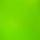Side of the square is a = 6.2 cm, how long is its diagonal?
• Square diagonalCalculate the length of the square diagonal if the perimeter is 476 cm.
• Diagonal of the rectangleCalculate the diagonal of the rectangle which area is 54 centimeters square and the circuit is equal to 30 cm.
• Wall diagonalCalculate the length of wall diagonal of the cube whose surface is 384 cm square.
• Space diagonalThe space diagonal of a cube is 129.91 mm. Find the lateral area, surface area and the volume of the cube.
• Square diagonalCalculate the length of diagonal of the square with side a = 23 cm.
• Body diagonal - cubeCalculate the surface and cube volume with body diagonal 15 cm long.
• Construct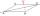Construct a rhombus ABCD, if the size of the diagonal AC is 6 cm and diagonal BD 8 cm long.
• Diagonal - simple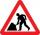Calculate the length of the diagonal of a rectangle with dimensions 5 cm and 12 cm.
• RhombusCalculate the length of the diagonal AC of the rhombus ABCD, if its perimeter is 84 dm and the other diagonal BD has length 20 dm.

Do you have an interesting mathematical word problem that you can't solve it? Submit a math problem, and we can try to solve it.

We will send a solution to your e-mail address. Solved examples are also published here. Please enter the e-mail correctly and check whether you don't have a full mailbox.

Please do not submit problems from current active competitions such as Mathematical Olympiad, correspondence seminars etc...

Pythagorean theorem is the base for the right triangle calculator.